Structural Bioinformatics Library Template C++ / Python API for developping structural bioinformatics applications.
User Manual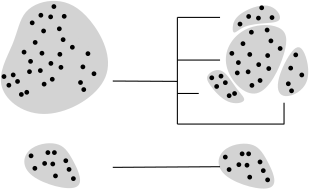# D_family_matching

Authors: R. Tetley and F. Cazals and D. Mazauric

# Introduction

While clustering is ubiquitous in data science, with selected methods provided in the package Cluster_engines, picking a particular clustering algorithm and tuning its parameters is in general a non trivial task.

In order to alleviate this task, this package provides methods to compare two clusterings, by computing a mapping between the clusters of these clusterings. In doing so, groups of clusters called meta-clusters are formed within each clustering, and a 1-to-1 correspondence between these meta-clusters is provided. (We note in passing that our mapping goes beyond a 1-to-1 matching between the clusters ,  .)

The following comments are in order:

• It has been noticed  that In fact, the right number of clusters in a dataset often depends on the scale at which the dataset is inspected. In this package, we provide algorithms which are parameterized by a scale parameter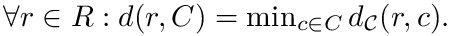, namely the maximum diameter of the subgraph connecting clusters to define meta-clusters.
• Various cluster comparison measures have been proposed, and we refer the reader to  for a thorough review of these. The main drawback of these methods are that (i) they typically return a number qualifying the coherence between the clusterings, without any mapping the clusters with one another, and (ii) they do not accommodate any scale parameter.

In the sequel, we formalize the clustering comparison problem in a manner answering the two needs just discussed, and document the classes provided. For comparison purposes, this package also provides an implementation of the variation of information (VI)  , a metric based on information theoretical concepts.

# Algorithms

## Terminology and pre-requisites

Consider a dataset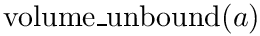consisting of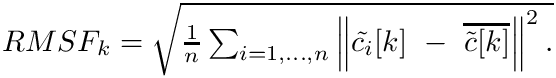observations (data points or points for short). A clustering is a partition of the input dataset into disjoint subsets called clusters.

A partial clustering is a collection of clusters whose union is strictly contained in the input dataset (e.g. some outliers have been removed).

Consider a datasetand two partial clusterings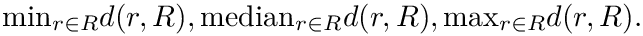and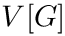of. The edge-weighted intersection graph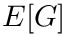is then constructed as follows. The set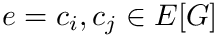corresponds to the clustering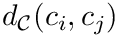. To each vertex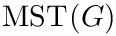, we associate the set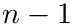. The set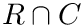corresponds to the clustering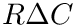. To each vertex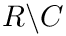, we associate the set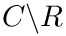. The set of edges of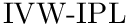is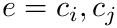. The weight of any edge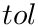is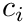. The number of edges and vertices of the intersection graph are respectively denoted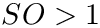and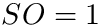.

Consider two clusteringsandof an identical dataset. To a given cluster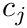we associate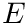whereand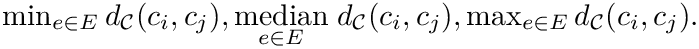. The entropy associated with clusteringis defined as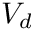. Let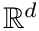and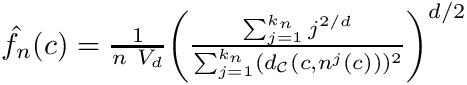, define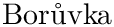. The mutual information betweenandis defined as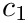. From this, we define the variation of information betweenandas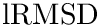.

## Problem statement

Let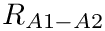. Let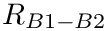be an intersection graph. A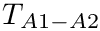foris a family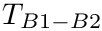, such that, for every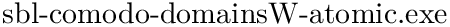, if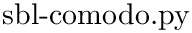, then: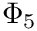and the graph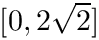induced by the set of nodes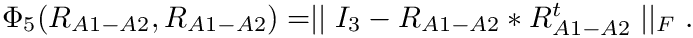has diameter at most.

The score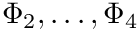of a-family-matching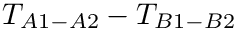is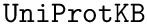.

Let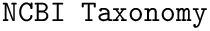be the set of all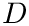for. Let. Given an intersection graph, the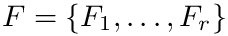consists in computing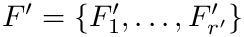}.

Intuitively, the D-family-matching problem involves computing disjoint subset of nodes (clusters of clusters, or meta-clusters) such that the inconsitencies are minimized (clusters which are in the same meta-cluster have a minimum number of divergent points).

## Problem hardness

The decision version of theproblem is NP-complete for:

• bipartite graphs of maximum degree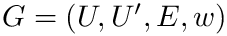• bipartite graphs of maximum degree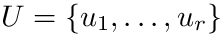even if the maximum weight is constant.

The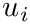problem is NP-complete for bipartite graphs of maximum degreeand with unary weights.

There exist polynomial time algorithms for certain classes of graphs. Denotingandthe number of vertices and edges of the intersection graph, the following is proved in  :

• For a tree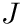, theproblem can be solved in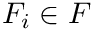time (where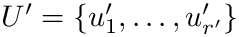is the maximum degree of).
• For a path, theproblem can be solved in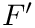time.
• For a cycle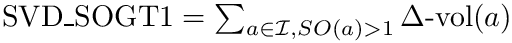(or any graph of maximum degree 2), theproblem can be solved in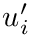time.

## Algorithms

The implemented algorithms follows the generic design presented in  .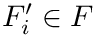We implemented a version of the previous algorithm called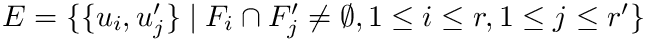(for Spanning Tree Solver):

• the spanning tree generator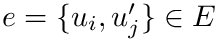returns a maximum spaning tree, or a random spanning tree ;
• the property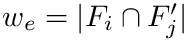returns true once we have computed a solution on the maximum spanning tree, as well as a solution ondistinct random spanning trees (for a given) or if the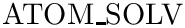first distinct solutions have a score contained in an interval of size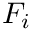.
•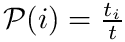is the polynomial time algorithm on trees described in  with an additional step: edges for which both extremities belong to the same meta-cluster (and previously unaccounted for) are added to the said meta-cluster.

# Implementation and functionalities

## Design

The package is centered around the generic the algorithm discussed above.

• We provide two module classes (for modules, see Module_base ): SBL::Modules::T_RST_MST_D_Family_matching_module< ModuleTraits > is thealgorithm packaged in a module, SBL::Modules::T_D_Family_matching_module< Graph , TSolutionComputer > lets the user decide which solution computer to use (can be a custom one or any of the ones provided).
• The class SBL::CADS::T_VI_computer< Clustering > provides a functor for computing the so called variation of information.

## Functionalities

• Generic input: two text files giving for each point the index of its clusters (as outputed by Cluster_engines)
• D-family-matching output: for each clustering, a .txt file mapping each original cluster to its meta-cluster, a .dot file containing the intersection graph as well as the solution highlighted in red, a .xml file containing various statistics on all the computed solutions (number of meta-clusters, score, size, etc...)
Visualization: we also provide scripts for displaying the meta-clusters on the original point clouds.

# Examples

This example loads two input clusterings and computes their 3-family matching using random spanning trees or a minimum spanning tree.

// An individual element of a cluster
struct Cluster_element
{
unsigned m_element_number;
Cluster_element(){};
Cluster_element(unsigned number) : m_element_number(number) {};
unsigned element_number() {return this->m_element_number; };
unsigned element_number() const {return this->m_element_number; };
template <class Archive>
void serialize(Archive &ar, const unsigned BOOST_PFTO int version)
{
ar & boost::serialization::make_nvp("element", this->m_element_number);
};
};
// A cluster type
typedef std::vector<Cluster_element> Cluster;
// A clustering
typedef std::vector<Cluster> Clustering;
// Property for the vertices of the graph
struct Vertex_property
{
//cluster associated with the vertex
Cluster* cluster;
//index of the cluster (in the given clustering)
unsigned cluster_index;
//index of the meta cluster
int meta_index;
//part of the graph which this vertex belongs to
unsigned part;
};
// The weights correspond to the size of the cluster intersections
typedef boost::property<boost::edge_weight_t, int> Edge_weight_property;
//Weighted graph of intersections
boost::vecS,
boost::undirectedS,
Vertex_property,
Edge_weight_property> Intersection_graph;
typedef typename RST_Solution_computer::Solution_type RST_Solution_type;
typedef typename MST_Solution_computer::Meta_clusters_iterator MST_meta_cluster_iterator;
typedef typename MST_Solution_computer::Clusters_iterator MST_cluster_iterator;
typedef typename RST_Solution_computer::Meta_clusters_iterator RST_meta_cluster_iterator;
typedef typename RST_Solution_computer::Clusters_iterator RST_cluster_iterator;
int main(int argc, char *argv[])
{
if (argc != 3)
{
std::cout << "Provide two clusterings for comparison!"<< std::endl;
std::exit(EXIT_FAILURE);
}
// load the clusterings and build the graph (done within the loader class)
// Build the MST solution computer and compute a solution
MST_Solution_type mst_solution;
MST_Solution_computer mst(g);
unsigned mst_score = mst(3, mst_solution);
// Build the RST solution computer and compute a solution
RST_Solution_type rst_solutions;
RST_Solution_computer rst(g);
unsigned rst_score = rst(3, rst_solutions);
std::cout << "MST 3-family matching: \nScore: " << mst_score << std::endl ;
std::cout << "Meta clusters: " << std::endl;
//Showcase the iterators
MST_meta_cluster_iterator mm_it;
MST_cluster_iterator mc_it;
for(mm_it = mst.meta_clusters_begin(); mm_it != mst.meta_clusters_end(); mm_it++)
{
mc_it = mst.clusters_in_meta_cluster_begin(*mm_it);
std::cout << *mm_it << " <==> {" << *mc_it;
mc_it++;
for(; mc_it != mst.clusters_in_meta_cluster_end(*mm_it); mc_it++)
std::cout << ", " << *mc_it;
std::cout << "}" << std::endl;
}
std::cout << "RST 3-family matching: \nScore: " << rst_score << std::endl;
std::cout << "Meta clusters: " << std::endl;
RST_meta_cluster_iterator rm_it;
RST_cluster_iterator rc_it;
for(rm_it = rst.meta_clusters_begin(); rm_it != rst.meta_clusters_end(); rm_it++)
{
rc_it = rst.clusters_in_meta_cluster_begin(*rm_it);
std::cout << *rm_it << " <==> {" << *rc_it;
rc_it++;
for(; rc_it != rst.clusters_in_meta_cluster_end(*rm_it); rc_it++)
std::cout << ", " << *rc_it;
std::cout << "}" << std::endl;
}
return 0;
}

# Applications

## Programs

This package provides two executables to compare clusterings:

• sbl-cmp-clust-VI.exe : computes the so-called Variation of Information.
• sbl-cmp-clust-dfam.exe : computes the meta-clusters from two input clusterings. It is a direct implementation of thealgorithm. This executable is described thereafter.

## Main specifications

Main options. The main options are:

The main options are: –filenames  string: Clustering filename – must be passed twice
–diameter-constraint  string: Max diameter for meta-cluster
–num-iterations  string: Num of iterations for random spanning trees

Input. The two input clusterings. Format specified in the package Cluster_engines .

Main output.

Consists of three files:

• Graph of metaclusters, dot format, suffix __graph.dot: the bipartite graph whose nodes are the clusters, and the connected components defined meta-clusters
• Metacluster file, txt format, suffix (clust1 | clust2) _meta_clusters.txt : the text file assigning clusters of the first clustering (resp. second clustering) to metaclusters.

# Jupyter demo

See the following jupyter notebook:

• Jupyter notebook file
• D_family_matching

# D_family_matching¶

## Useful functions¶

In :
from SBL import sbl_jupyter as sblj
help(sblj)

Help on module SBL.sbl_jupyter in SBL:

NAME
SBL.sbl_jupyter

DESCRIPTION
#from SBL import sbl_jupyter as sblj
#help(sblj)
#
## Use as follows
# sblj.SBLjupyter.find_and_show_images(".png", odir, 50)

CLASSES
builtins.object
tools

class tools(builtins.object)
|  Static methods defined here:
|
|  convert_eps_to_png(ifname, osize)
|
|  convert_pdf_to_png(ifname, osize)
|
|  find_and_convert(suffix, odir, osize)
|      # find file with suffix, convert, and return image file
|
|  find_and_show_images(suffix, odir, osize)
|
|  find_file_in_output_directory(suffix, odir)
|
|  show_eps_file(eps_file, osize)
|
|  show_image(img)
|
|  show_log_file(odir)
|
|  show_pdf_file(pdf_file)
|
|  show_row_n_images(images, size)
|
|  ----------------------------------------------------------------------
|  Data descriptors defined here:
|
|  __dict__
|      dictionary for instance variables (if defined)
|
|  __weakref__
|      list of weak references to the object (if defined)

FILE
/user/fcazals/home/projects/proj-soft/sbl/python/SBL/sbl_jupyter.py



## Toy example: comparing two clusters¶

### Options.¶

We provide a simple example using the executable sbl-cmp-clust-dfam.exe.

The main options of the cmpClusters in the next cell are:

• cluster1: Path to the first cluster file
• cluster2: Path to the second second cluster file
• method: method (dfam or VI)
• dfam: using the D_family_matching
• VI: using the Variation of Information
In :
import re  #regular expressions
import sys #misc system
import os
import pdb
import shutil # python 3 only

import matplotlib.pyplot as plt
import matplotlib.image as mplimg

def cmp_clusters(cluster1, cluster2, method = "dfam"):

odir = "results-toy-%s" % method
if os.path.exists(odir):
os.system("rm -rf %s" % odir)
os.system( ("mkdir %s" % odir) )

# check executable exists and is visible
exe = shutil.which("sbl-cmp-clust-%s.exe" % method)
if exe:
print(("Using executable %s\n" % exe))
cmd = "sbl-cmp-clust-%s.exe -f %s -f %s -d %s --log" % (method, cluster1, cluster2,odir)
print(cmd)
os.system(cmd)

cmd = "ls %s/*" % odir
print("All output files:",ofiles)

# we also generate the graph connecting clusters withig meta-clusters, see graph below
if(method=="dfam"):
cmd = "dot -Tpdf %s/sbl-d-family-matching__graph.dot -o %s/graph.pdf" % (odir,odir)
os.system(cmd)
sblj.tools.convert_pdf_to_png( ("%s/graph.pdf" % odir), 150 )
plt.xticks([]); plt.yticks([])
imgplot = plt.imshow(img)

#find the log file and display log file
#log = open( ("%s/log.txt") % odir).readlines()
#for line in log:         print(line.rstrip())

else:


In :
print("Marker : Calculation Started")
#cmp_clusters("data/cluster_1.txt","data/cluster_2.txt", "VI")
cmp_clusters("data/cluster_1.txt","data/cluster_2.txt")
print("Marker : Calculation Ended")

Marker : Calculation Started
Using executable /user/fcazals/home/projects/proj-soft/sbl-install/bin/sbl-cmp-clust-dfam.exe

sbl-cmp-clust-dfam.exe -f data/cluster_1.txt -f data/cluster_2.txt -d results-toy-dfam --log
All output files: ['results-toy-dfam/sbl-d-family-matching__clust1_meta_clusters.txt\n', 'results-toy-dfam/sbl-d-family-matching__clust2_meta_clusters.txt\n', 'results-toy-dfam/sbl-d-family-matching__graph.dot\n', 'results-toy-dfam/sbl-d-family-matching__k_family_matching.xml\n', 'results-toy-dfam/sbl-d-family-matching__log.txt\n']
Marker : Calculation Ended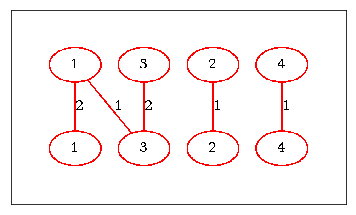## Comparing clusterings produced by kmeans¶

In the following, we use sbl-cmp-clust-dfam.exe to compare clusterings produced by kmeans. See the package Clustering_engines for the clustering algorithms.

### In the following, we perform the following steps:¶

• Step 1: prepare the individual clusterings of the datasets
• Step 1b: plot clusterings and collect the images
• Step 2: compute the D-family matchig with the constraint D_max given
• Step 3: create a clustering file from the meta-cluster mapping and the original cluster file
• Step 4: display all results
• Step 5 : extract statistics from xml output file with PALSE
In :
import os
import pdb
import sys

from SBL import PALSE
from PALSE import *

#cluster the input data (contained in the .txt file) set into 5 cluster using k-means++
points_files = ["data/points-N200-d50.txt", "data/points-N200-d50.txt"]
k_values = [10, 20]

def run_with_diameter_constraint(D_max):

odir = "results-%s" % D_max
if os.path.exists(odir):
os.system("rm -rf %s" % odir)
os.system( ("mkdir %s" % odir) )

# Step 1: prepare the individual clusterings of the datasets
clusters_files = [] # files containing cluters
centers_files = [] # files containing cluster centers
for i in range(0, len(points_files)):
cmd = "sbl-cluster-k-means-euclid.exe --k-means-selector=plusplus\
--points-file %s --k-means-k %d -o -d %s" % (points_files[i], k_values[i], odir)
print("Executing ",cmd)
os.system(cmd)

cmd = "find %s -name \"*k_%s__clusters.txt\"" % (odir, k_values[i])

cmd = "find %s  -name \"*k_%s__centers.txt\"" % (odir, k_values[i])

# Step 1b: plot clusterings and collect the images
for i in range(0, len(points_files)):
cmd = "sbl-clusters-display.py -f %s -c %s -C %s -o %s" % (points_files[i], clusters_files[i], centers_files[i], odir)
os.system(cmd)

# collect the plots
cmd = "find %s -name \"*centers.png\"" % odir
images = [line.rstrip() for line in lines]

# Step 2: compute the D-family matchig with the constraint D_max given
# Nb: we use 100 iterations by default.
cmd = "sbl-cmp-clust-dfam.exe -f %s -f %s --diameter-constraint %d --num-iterations 100 -d %s" % (clusters_files, clusters_files, D_max, odir)
print("Clustering comparison command:",cmd)
os.system(cmd)

# Step 3: create a clustering file from the meta-cluster mapping and the original cluster file
cmd = "find %s -name \"*clust1_meta_clusters.txt\"" % odir
#print(meta_cluster_file)

cmd = "sbl-map-meta-clusters.py  -c %s -m %s  -o %s/final-clustering.txt" % (clusters_files, meta_cluster_file,odir)
os.system(cmd)

cmd = "sbl-clusters-display.py  -f %s -c %s/final-clustering.txt -o %s" % (points_files,odir,odir)
print("Cluster display command for meta clustering:",cmd)
os.system(cmd)

# Step 4: display all results
cmd = "find %s  -name \"*final-clustering.png\"" % odir

images.append(final_png)
sblj.tools.show_row_n_images(images, 50)

# Step 5 : palse
database = PALSE_xml_DB()

# total score
score = database.get_all_data_values_from_database("d_family_matching/solution/best_solution/score", int)

# stats on meta-clusters
meta_cluster_sizes = database.get_all_data_values_from_database("d_family_matching/solution/best_solution/meta_cluster_sizes/item", int)
print("Score of the matching:",score)
print("Num meta clusters:", len(meta_cluster_sizes))
print("Num of clusters in each meta-clusters:",meta_cluster_sizes)

return odir



### We now run the previous function for increasing values of D_max.¶

In particular, we analyze the incidence of the maximum diameter constraint. Given that the original input file contains 1000 points, note that the total score (num. of points found in meta-clusters) converges rapidly to the maximum possible balue when increasing D_max.

NB: caption for figures:

• left plot: first clustering
• middle plot: second clustering
• right plot: meta-clustering
In :
run_with_diameter_constraint(2)

Executing  sbl-cluster-k-means-euclid.exe --k-means-selector=plusplus              --points-file data/points-N200-d50.txt --k-means-k 10 -o -d results-2
Executing  sbl-cluster-k-means-euclid.exe --k-means-selector=plusplus              --points-file data/points-N200-d50.txt --k-means-k 20 -o -d results-2
Clustering comparison command: sbl-cmp-clust-dfam.exe -f results-2/sbl-cluster-k-means-euclid__f_points-N200-d50__mode_plusplus__iter_max_10__k_10__clusters.txt -f results-2/sbl-cluster-k-means-euclid__f_points-N200-d50__mode_plusplus__iter_max_10__k_20__clusters.txt --diameter-constraint 2 --num-iterations 100 -d results-2
Cluster display command for meta clustering: sbl-clusters-display.py  -f data/points-N200-d50.txt -c results-2/final-clustering.txt -o results-2
Figs displayed
XML: 1 / 1 files were loaded

Score of the matching: 786
Num meta clusters: 9
Num of clusters in each meta-clusters: [4, 3, 3, 3, 3, 3, 2, 4, 5]

Out:
'results-2'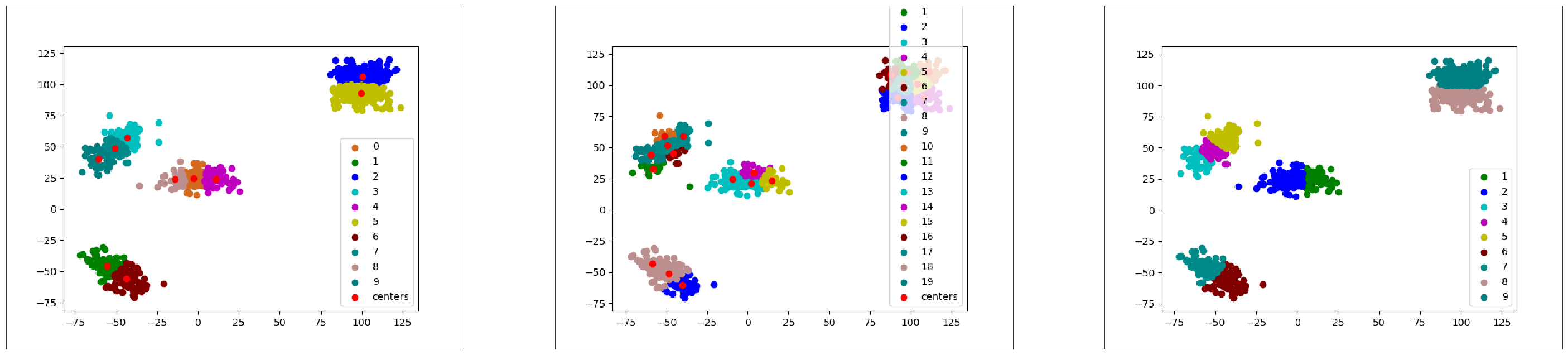In :
run_with_diameter_constraint(4)

Executing  sbl-cluster-k-means-euclid.exe --k-means-selector=plusplus              --points-file data/points-N200-d50.txt --k-means-k 10 -o -d results-4
Executing  sbl-cluster-k-means-euclid.exe --k-means-selector=plusplus              --points-file data/points-N200-d50.txt --k-means-k 20 -o -d results-4
Clustering comparison command: sbl-cmp-clust-dfam.exe -f results-4/sbl-cluster-k-means-euclid__f_points-N200-d50__mode_plusplus__iter_max_10__k_10__clusters.txt -f results-4/sbl-cluster-k-means-euclid__f_points-N200-d50__mode_plusplus__iter_max_10__k_20__clusters.txt --diameter-constraint 4 --num-iterations 100 -d results-4
Cluster display command for meta clustering: sbl-clusters-display.py  -f data/points-N200-d50.txt -c results-4/final-clustering.txt -o results-4
Figs displayed
XML: 1 / 1 files were loaded

Score of the matching: 956
Num meta clusters: 6
Num of clusters in each meta-clusters: [4, 6, 9, 3, 3, 5]

Out:
'results-4'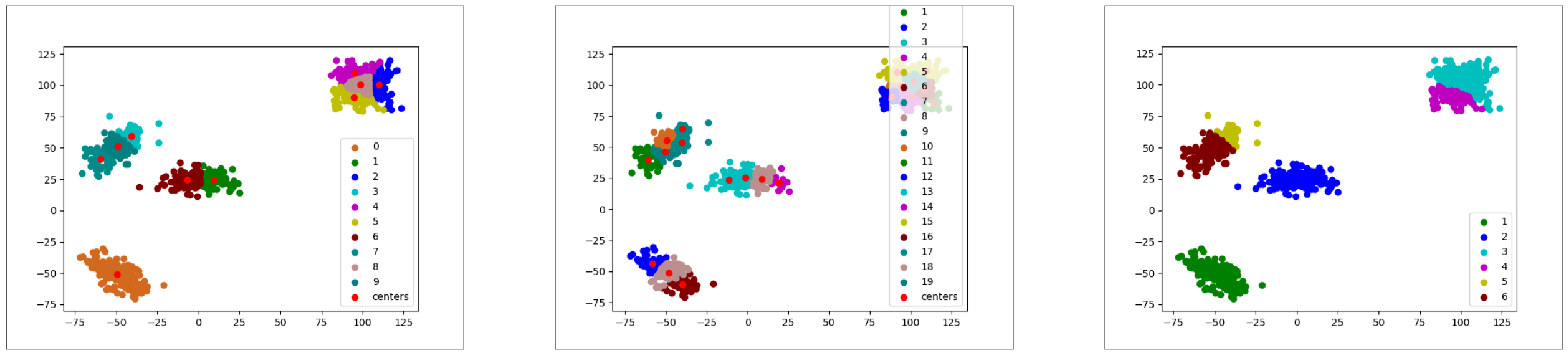In :
run_with_diameter_constraint(8)

Executing  sbl-cluster-k-means-euclid.exe --k-means-selector=plusplus              --points-file data/points-N200-d50.txt --k-means-k 10 -o -d results-8
Executing  sbl-cluster-k-means-euclid.exe --k-means-selector=plusplus              --points-file data/points-N200-d50.txt --k-means-k 20 -o -d results-8
Clustering comparison command: sbl-cmp-clust-dfam.exe -f results-8/sbl-cluster-k-means-euclid__f_points-N200-d50__mode_plusplus__iter_max_10__k_10__clusters.txt -f results-8/sbl-cluster-k-means-euclid__f_points-N200-d50__mode_plusplus__iter_max_10__k_20__clusters.txt --diameter-constraint 8 --num-iterations 100 -d results-8
Cluster display command for meta clustering: sbl-clusters-display.py  -f data/points-N200-d50.txt -c results-8/final-clustering.txt -o results-8
Figs displayed
XML: 1 / 1 files were loaded

Score of the matching: 1000
Num meta clusters: 4
Num of clusters in each meta-clusters: [5, 6, 11, 8]

Out:
'results-8'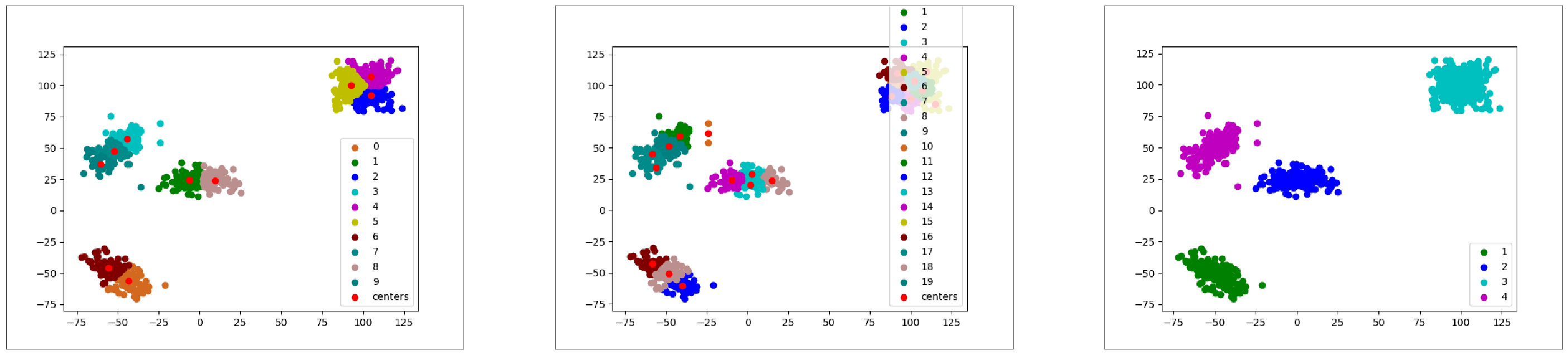The reader is referred to  for a detailed comparison of VI against the scores yielded by our comparison strategy.